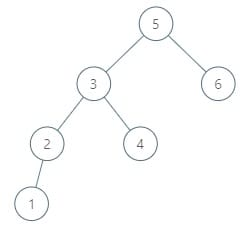# 285. Inorder Successor in BST

Reference: LeetCode
Difficulty: Medium

## Problem

Given a binary search tree and a node in it, find the in-order successor of that node in the BST. The successor of a node p is the node with the smallest key greater than p.val.

Note:

• If the given node has no in-order successor in the tree, return null.
• It’s guaranteed that the values of the tree are unique.

Example:

## AnalysisMethods:

x is the current node; p is the target node.

1. Recursion
• If p is in the left subtree, the successor could be x or successor(x.left). It means that x is a possible candidate. It turns to be a real successor when the return value of successor(x.left) equals null.
• If p is in the right subtree, the successor must be in the right subtree if it exists.
• If p is found, we still go to right subtree to find its successor if it exists.
• The base case is when x reaches null.
• Time: $O(h)$
• Space: $O(h)$
2. Iteration
• We use a variable succ to record the successor, which is initially null.
• If p.val is less than x.val, it needs to go left, and we need to update succ.
• If p.val is greater than or equal to x.val:
• greater than: Just go right. x.left and x couldn’t be the successor.
• equal to: Just go right too. In the next round, p.val will be less than x.val, it will set the succ to the would-be right child x.
• Time: $O(h)$
• Space: $O(1)$

## Code

Test Case:

Predecessor:

### Ceil

Code: algs4 - BST.java

The idea is also applied to ceil operation:

CommentJunhao Wang
a software engineering cat